# GMAT Math : DSQ: Calculating an angle in a polygon

## Example Questions

← Previous 1

### Example Question #9 : Polygons

How many sides does a regular polygon have?

1) Each of the angles measures 140 degrees.

2) Each of the sides has measure 8.

Possible Answers:

BOTH statements TOGETHER are sufficient to answer the question, but NEITHER statement ALONE is sufficient to answer the question.

BOTH statements TOGETHER are NOT sufficient to answer the question.

Statement 2 ALONE is sufficient to answer the question, but Statement 1 ALONE is not sufficient.

Statement 1 ALONE is sufficient to answer the question, but Statement 2 ALONE is not sufficient.

EITHER Statement 1 or Statement 2 ALONE is sufficient to answer the question.

Correct answer:

Statement 1 ALONE is sufficient to answer the question, but Statement 2 ALONE is not sufficient.

Explanation:

The relationship between the number of sides of a regular polygonand the measure of a single angleis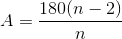If we are given that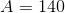, then we can substitute and solve for: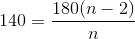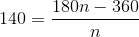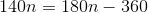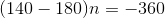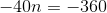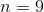making the figure a nine-sided polygon.

Knowing only the measure of each side is neither necessary nor helpful; for example, it is possible to construct an equilateral triangle with sidelength 8 or a square with sidelength 8.

The correct answer is that Statement 1 alone is sufficient to answer the question, but not Statement 2 alone.

### Example Question #10 : Polygons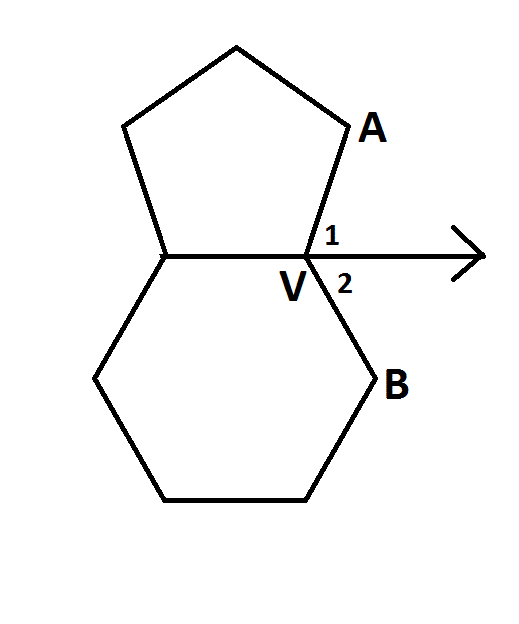Note: Figure NOT drawn to scale.

Is the pentagon in the diagram above a regular pentagon?

Statement 1: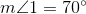Statement 2: The hexagon in the diagram is not a regular hexagon.

Possible Answers:

BOTH statements TOGETHER are sufficient to answer the question, but NEITHER statement ALONE is sufficient to answer the question.

EITHER statement ALONE is sufficient to answer the question.

BOTH statements TOGETHER are insufficient to answer the question.

Statement 2 ALONE is sufficient to answer the question, but Statement 1 ALONE is NOT sufficient to answer the question.

Statement 1 ALONE is sufficient to answer the question, but Statement 2 ALONE is NOT sufficient to answer the question.

Correct answer:

Statement 1 ALONE is sufficient to answer the question, but Statement 2 ALONE is NOT sufficient to answer the question.

Explanation:

Information about the hexagon is irrelevant, so Statement 2 has no bearing on the answer to the question.

The measures of the exterior angles of any pentagon, one per vertex, total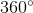, and they are congruent if the pentagon is regular, so if this is the case, each would measure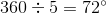. But if Statement 1 is true, then an exterior angle of the pentagon measures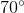. Therefore, Statement 1 is enough to answer the question in the negative.

### Example Question #1 : Dsq: Calculating An Angle In A Polygon

What is the sum of six numbers,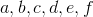?

Statement 1:are the measures of the interior angles of a hexagon.

Statement 2: The median of the data set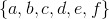is 120.

Possible Answers:

EITHER statement ALONE is sufficient to answer the question.

Statement 1 ALONE is sufficient to answer the question, but Statement 2 ALONE is NOT sufficient to answer the question.

BOTH statements TOGETHER are sufficient to answer the question, but NEITHER statement ALONE is sufficient to answer the question.

BOTH statements TOGETHER are insufficient to answer the question.

Statement 2 ALONE is sufficient to answer the question, but Statement 1 ALONE is NOT sufficient to answer the question.

Correct answer:

Statement 1 ALONE is sufficient to answer the question, but Statement 2 ALONE is NOT sufficient to answer the question.

Explanation:

The sum of the measures of the six interior angles of any hexagon is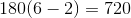, so if Statement 1 is true, then the sum of the six numbers is 720, regardless of the individual values.

Statement 2 is not enough, however, for us to deduce this sum; this only tells us the mean of the third- and fourth-highest values.

### Example Question #12 : Polygons

What is the sum of five numbers,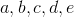?

Statement 1:are the measures of the interior angles of a pentagon.

Statement 2: The mean of the data set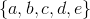is 108.

Possible Answers:

BOTH statements TOGETHER are sufficient to answer the question, but NEITHER statement ALONE is sufficient to answer the question.

EITHER statement ALONE is sufficient to answer the question.

Statement 2 ALONE is sufficient to answer the question, but Statement 1 ALONE is NOT sufficient to answer the question.

BOTH statements TOGETHER are insufficient to answer the question.

Statement 1 ALONE is sufficient to answer the question, but Statement 2 ALONE is NOT sufficient to answer the question.

Correct answer:

EITHER statement ALONE is sufficient to answer the question.

Explanation:

The sum of the measures of the five interior angles of any pentagon is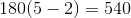, so if Statement 1 is true, then the sum of the five numbers is 540, regardless of the individual values.

The mean of five data values multiplied by 5 is the sum of the values, so if Statement 2 is true, then their sum is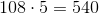.

### Example Question #13 : Polygons

What is the measure of an interior angle of a regular polygon?

Statement 1: The polygon has 20 sides.

Statement 2: An exterior angle of the polygon measures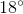.

Possible Answers:

BOTH statements TOGETHER are sufficient to answer the question, but NEITHER statement ALONE is sufficient to answer the question.

Statement 1 ALONE is sufficient to answer the question, but Statement 2 ALONE is NOT sufficient to answer the question.

Statement 2 ALONE is sufficient to answer the question, but Statement 1 ALONE is NOT sufficient to answer the question.

BOTH statements TOGETHER are insufficient to answer the question.

EITHER statement ALONE is sufficient to answer the question.

Correct answer:

EITHER statement ALONE is sufficient to answer the question.

Explanation:

From Statement 1, you can calculate the measure of an interior angle as follows: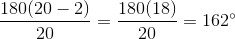From Statement 2, since an interior angle and an exterior angle at the same vertex form a linear pair, they are supplementary, so you can subtract 18 from 180: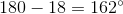### Example Question #14 : Polygons

Is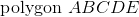a regular pentagon?

Statement 1: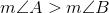Statement 2: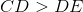Possible Answers:

Statement 2 ALONE is sufficient to answer the question, but Statement 1 ALONE is NOT sufficient to answer the question.

EITHER statement ALONE is sufficient to answer the question.

BOTH statements TOGETHER are insufficient to answer the question.

BOTH statements TOGETHER are sufficient to answer the question, but NEITHER statement ALONE is sufficient to answer the question.

Statement 1 ALONE is sufficient to answer the question, but Statement 2 ALONE is NOT sufficient to answer the question.

Correct answer:

EITHER statement ALONE is sufficient to answer the question.

Explanation:

All of the interior angles of a regular polygon are congruent, as are all of its sides. Statement 1 violates the former condition; statement 2 violates the latter.

### Example Question #15 : Polygons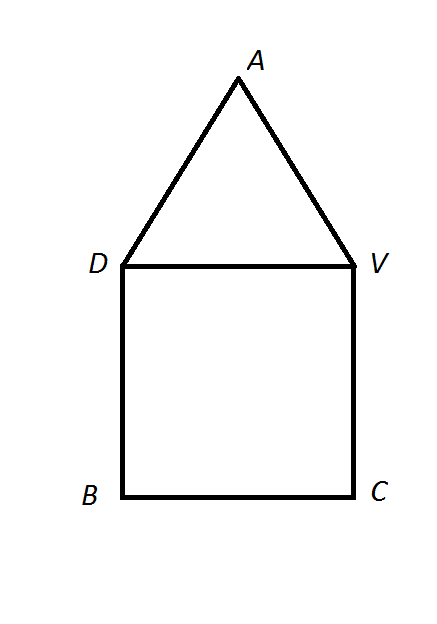Note: Figure NOT drawn to scale.

The diagram above shows a triangle and a rhombus sharing a side. Is that rhombus a square?

Statement 1: The triangle is not equilateral.

Statement 2: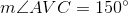Possible Answers:

BOTH statements TOGETHER are insufficient to answer the question.

Statement 1 ALONE is sufficient to answer the question, but Statement 2 ALONE is NOT sufficient to answer the question.

Statement 2 ALONE is sufficient to answer the question, but Statement 1 ALONE is NOT sufficient to answer the question.

EITHER statement ALONE is sufficient to answer the question.

BOTH statements TOGETHER are sufficient to answer the question, but NEITHER statement ALONE is sufficient to answer the question.

Correct answer:

BOTH statements TOGETHER are insufficient to answer the question.

Explanation:

To show that the rhombus is a square, you need to demonstrate that one of its angles is a right angle - that is,. Both statements together are insufficent - if, you would need to demonstrate that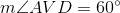is true or false, and the fact that the triangle is not equilateral is not enough to prove or to disprove this.

### Example Question #16 : Polygons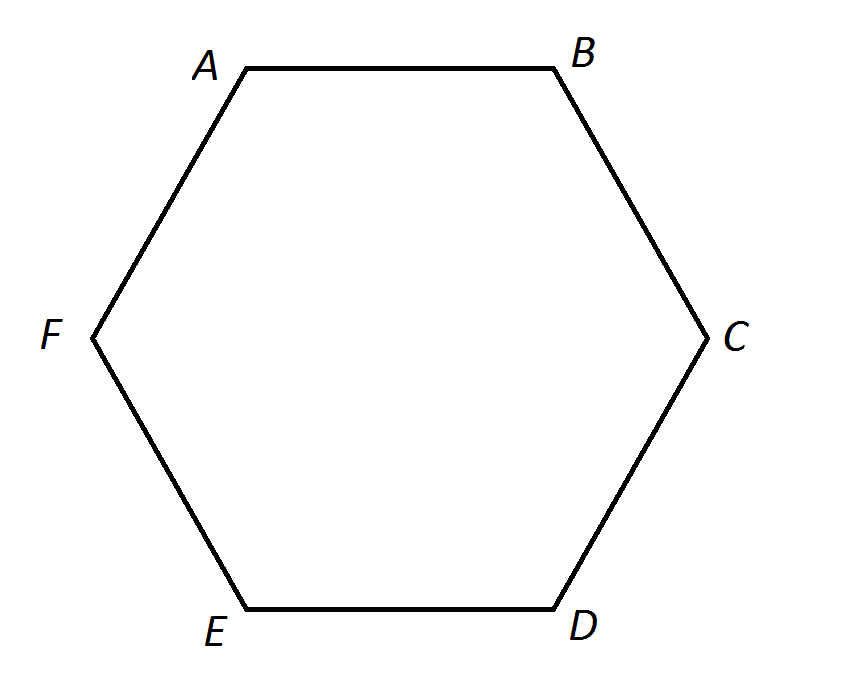Note: Figure NOT drawn to scale.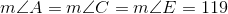What is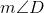?

Statement 1: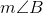, and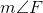are the first three terms, in order, of an arithmetic sequence.

Statement 2: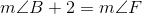Possible Answers:

Statement 1 ALONE is sufficient to answer the question, but Statement 2 ALONE is NOT sufficient to answer the question.

Statement 2 ALONE is sufficient to answer the question, but Statement 1 ALONE is NOT sufficient to answer the question.

EITHER statement ALONE is sufficient to answer the question.

BOTH statements TOGETHER are sufficient to answer the question, but NEITHER statement ALONE is sufficient to answer the question.

BOTH statements TOGETHER are insufficient to answer the question.

Correct answer:

Statement 1 ALONE is sufficient to answer the question, but Statement 2 ALONE is NOT sufficient to answer the question.

Explanation:

The sum of the measures of the angles of a hexagon is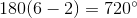Therefore,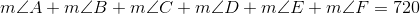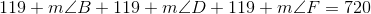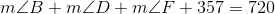Suppose we only know that, andare the first three terms of an arithmetic sequence, in order. Then for some common difference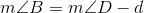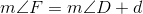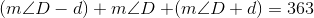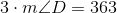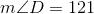Suppose we only know that. Then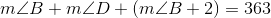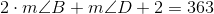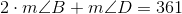With no further information, we cannot determine.

### Example Question #17 : Polygons

Is the degree measure of an exterior angle of a regular polygon an integer?

Statement 1: The number of sides of the polygon is a factor of 30.

Statement 2: The number of sides of the polygon is a factor of 40.

Possible Answers:

EITHER statement ALONE is sufficient to answer the question.

Statement 2 ALONE is sufficient to answer the question, but Statement 1 ALONE is NOT sufficient to answer the question.

BOTH statements TOGETHER are insufficient to answer the question.

BOTH statements TOGETHER are sufficient to answer the question, but NEITHER statement ALONE is sufficient to answer the question.

Statement 1 ALONE is sufficient to answer the question, but Statement 2 ALONE is NOT sufficient to answer the question.

Correct answer:

EITHER statement ALONE is sufficient to answer the question.

Explanation:

The sum of the degree measures of the exterior angles, one per vertex, of any polygon is 360, so each exterior angle of a regular polygon withsides measures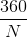. Therefore, the measure of one exterior angle of a regular polygon is an integer if and only ifis a factor of 360.

Ifis a factor of a factor of 360, however, thenis a factor of 360. 30 and 40 are both factors of 360: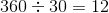and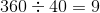. Therefore, it follows from either statement that the number of sides is a factor of 360, and each exterior angle has a degree measure that is an integer.

### Example Question #18 : Polygons

Is the degree measure of an exterior angle of a regular polygon an integer?

Statement 1: The number of sides of the polygon is divisible by 7.

Statement 2: The number of sides of the polygon is divisible by 10.

Possible Answers:

BOTH statements TOGETHER are sufficient to answer the question, but NEITHER statement ALONE is sufficient to answer the question.

Statement 2 ALONE is sufficient to answer the question, but Statement 1 ALONE is NOT sufficient to answer the question.

Statement 1 ALONE is sufficient to answer the question, but Statement 2 ALONE is NOT sufficient to answer the question.

EITHER statement ALONE is sufficient to answer the question.

BOTH statements TOGETHER are insufficient to answer the question.

Correct answer:

Statement 1 ALONE is sufficient to answer the question, but Statement 2 ALONE is NOT sufficient to answer the question.

Explanation:

The sum of the degree measures of the exterior angles, one per vertex, of any polygon is 360, so each exterior angle of a regular polygon withsides measuresForto be an integer, every factor ofmust be a factor of 360. This does not happen if 7 is a factor of, so Statement 1 disproves this. This may or may not happen if 10 is a factor of-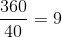, but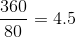, so Statement 2 does not provide an answer.

← Previous 1

### All GMAT Math Resources